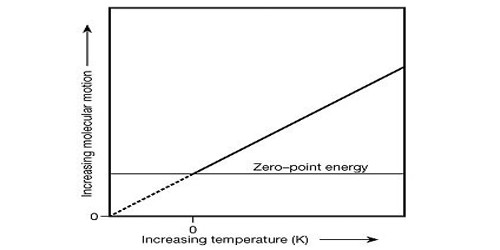Chemistry

# Thermodynamic TemperatureThermodynamic temperature is the absolute measure of temperature and is one of the principal parameters of thermodynamics. It is such that zero temperature is absolute zero (unlike Celsius, where -273.15°C is absolute zero). It is, therefore, a physical quantity that can be expressed in units, termed kelvin.

Thermodynamic temperature is defined by the third law of thermodynamics in which the theoretically lowest temperature is the null or zero points. The zero of the scale is absolute zero. The temperature of the ice point (0 °C) is 273.15kelvin. At this point, absolute zero, the particle constituents of matter have minimal motion and can become no colder. We generally don’t use “temperature” in thermodynamics, the thermodynamic temperature is more convenient. In the quantum-mechanical description, matter at absolute zero is in its ground state, which is its state of lowest energy. Thermodynamic temperature is often also called absolute temperature, for two reasons: the first, proposed by Kelvin, that it does not depend on the properties of a particular material; the second, that it refers to an absolute zero according to the properties of the ideal gas. It is expressed in a number of kelvins above absolute zero, the theoretical point at which nothing can get colder.

Thermodynamic temperature can be converted to Celsius temperature by subtracting 273.15 from the thermodynamic temperature. The International System of Units specifies a particular scale for thermodynamic temperature. It uses the Kelvin scale for measurement and selects the triple point of water at 273.16 K as the fundamental fixing point. Thus, internal energy and temperature are different, though directly related. Other scales have been in use historically. The Rankine scale, using the degree Fahrenheit as its unit interval, is still in use as part of the English Engineering Units in the United States in some engineering fields. The SI unit of energy is the joule. A “derived” SI unit, the joule itself is defined in terms of three SI base units — the kilogram, the meter and the second. ITS-90 gives a practical means of estimating the thermodynamic temperature to a very high degree of accuracy. But thermodynamic temperature is expressed in kelvins. There needs to be a way to connect the two.

Thermodynamic temperature is proportional to the average of all the energies in all the ways in which it is possible for an object to move. Roughly, the temperature of a body at rest is a measure of the mean of the energy of the translational, vibrational and rotational motions of matter’s particle constituents, such as molecules, atoms, and subatomic particles. Internal energy is loosely called the heat energy or thermal energy in conditions when no work is done upon the substance by its surroundings, or by the substance upon the surroundings. Internal energy may be stored in a number of ways within a substance, each way constituting a “degree of freedom”. Thermodynamic temperature can be converted to temperature on the Celsius scale by subtracting 273.15.